﻿ Deformation of Spring PlateExamples | Product | Murata Software Co., Ltd.Example6Deformation of Spring Plate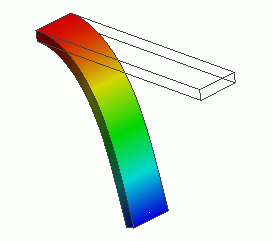General

• The deformation of a spring plate under forced displacement is analyzed.

• If an object is deformed beyond the limit of its elasticity, the large deformation occurs. It is treated as a nonlinear problem.
See Technical Note Analysis of Large Deformation (Geometric Nonlinearity).

• The distributions of displacement and mechanical stress are solved.

• Unless specified in the list below, the default conditions will be applied.

Analysis Space

 Item Settings Analysis Space 3D Model unit m

Analysis Conditions

This is a static analysis.
Select the “Large displacement” option.

This will activate Galileo’s nonlinear solver.

 Item Settings Solvers Mechanical Stress Analysis [Galileo] Analysis Type Static analysis Large deformation Select “Large displacement”.

Model

The spring plate is a rectangular solid body. The material is polycarbonate.

The plate is mechanically fixed at one end.

The forced displacement is applied on the other end.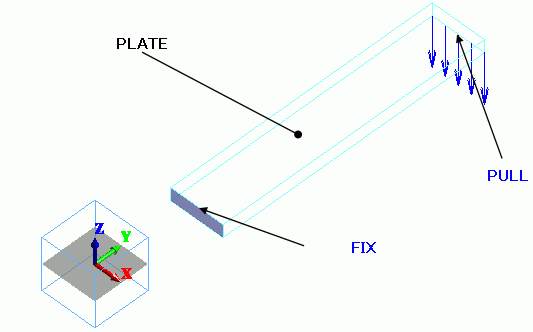Body Attributes and Materials

 Body Number/Type Body Attribute Name Material Name 0/Solid PLATE 000_Polyethylene(PE) *

* Available from the Material DB

Boundary Conditions

 Boundary Condition Name/Topology Tab Boundary Condition Type Settings FIX/Face Mechanical Displacement Select all X/Y/Z components. UX=0, UY=0, UZ=0 PULL/Edge Mechanical Displacement Select the Z component. Z=-15[m]

Results

The simulation is divided in 20 substeps. The displacement becomes larger gradually in the steps.

20 results are output for the steps between 0.05[Step] and 1[Step].

The number of substeps is 20 by default. It can be adjusted on the Step/Thermal Load tab.

All the results of substeps are output by default. Only final result of 1[Step] can be output too.

The displacement at 1[Step] is shown below. The contours are the Z displacement.

The scale adjustment ratio on the Displacement tab of the Graphics Setup is set to 1.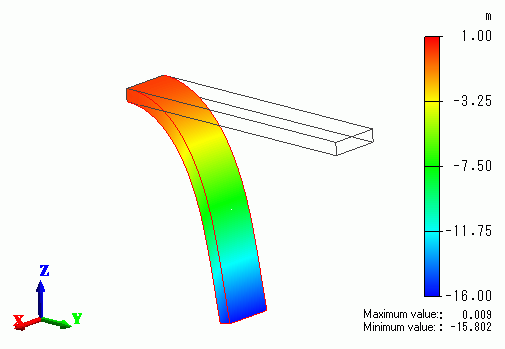The tip of the plate is curling towards -Y direction.

If [Show all results summary] is selected, the external force and reactive force are listed in the table below.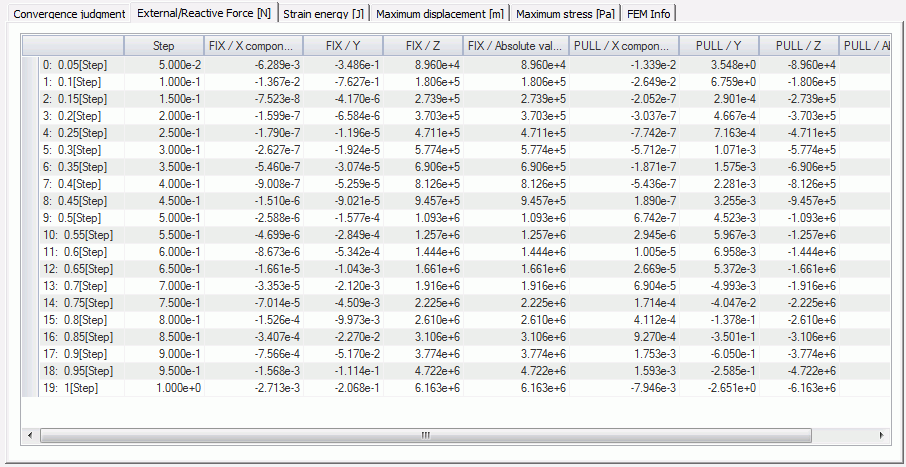The reactive force at the boundary condition FIX is 6.163e+6[N] in + Z direction at 1[Step].

The external force at PULL is 6.163e+6[N] in -Z direction at 1[Step].

For comparison, the displacement without Large displacement is calculated and the result is shown below.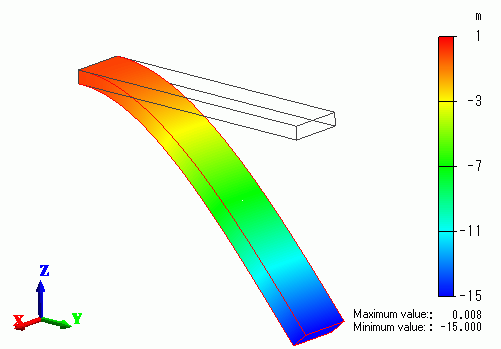The tip is not curling up. That is unnatural.

﻿